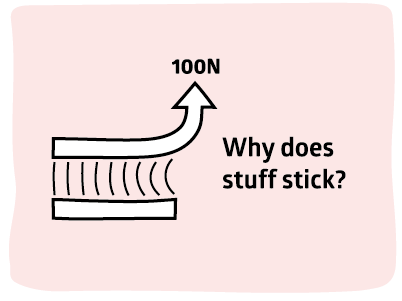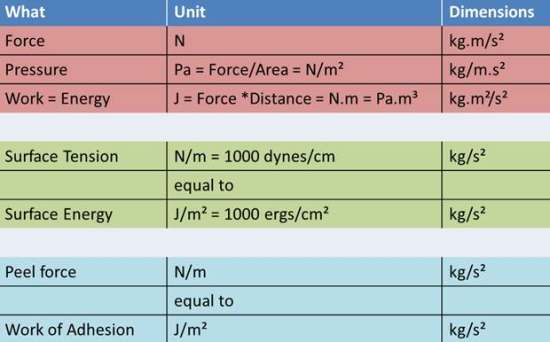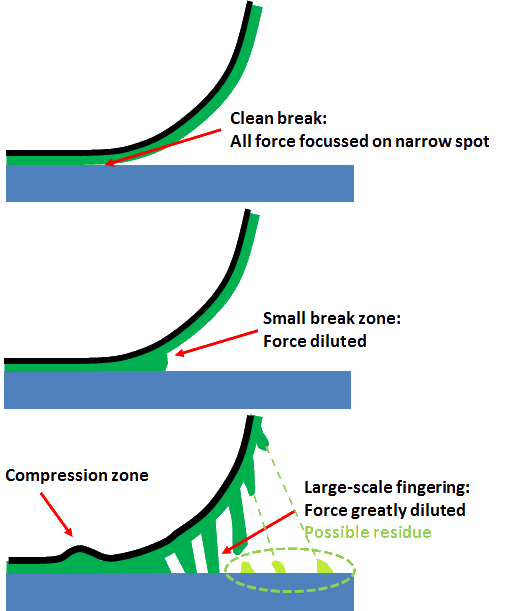## Why does stuff stick?If two surfaces separate it requires work to pull them apart. When the forces are due to surface energy, this work is labelled W, the Work of Adhesion. In general W is trivially small, less than 0.1J/m2, making it useless for most adhesive purposes. In other words, surface energy is largely irrelevant for practical adhesion unless you are a gecko. When the forces are more powerful we talk of G rather than W. For a typical "good" adhesive, G~100J/m2, i.e. 1000x larger than the adhesion from surface energy. One aim of this guide is to make it easy for you to understand what takes you from 0.1J/m2 to 100J/m2. The difference between W and G is sometimes called "adhesion hysteresis". This makes sense when the differences are modest (0.1-0.5J/m2) but the term isn't helpful for "real" adhesion when the W is a negligible part of the overall effect.

For simplicity, whether we have W or G, the calculated value shown in the modeller is always referred to as Work of Adhesion in J/m2.

Because units can be confusing here is a helpful table:So note that Peel force (the force, in N, needed to pull things apart along a line, in m, so N/m) is the same as the Work of Adhesion (the work, or energy, in J, needed to pull apart a whole area, in m2, so J/m2) because:

Work (Joules) = Force (N) * Distance_Moved (m) = N * m
so
J/m2 = N*m/m2 = N/m

In fact, according to basic theory, for a 90° pull, P=W but for a 180° pull W=2P [W=P*(1-cosθ)].

### Three types of failure